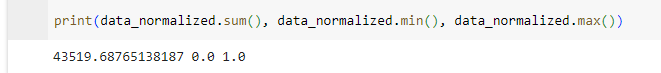# Mixture of Beta Distribution: Cannot find valid initial parameters

Hello everyone,

While in my previous thread I solved the same error with Gamma mixtures, I am super out of ideas with Beta mixture. I already normalized the data to absolutely [0,1]. Moreover, I do not know if it’s necessary but I kept the alpha and beta values rounded to 2 decimal places.

The interesting thing is:

1. the data had size (120000,) which did not work.
2. But subset of (1000,) worked.
3. other larger sizes did not work.
4. And now after few tries, even size of (1000,) stopped working…

Any tips or suggestions are greatly appreciated! Thank you all in advance!!

``````@config_enumerate
def beta_mixture(data, K):

data_min = data.min()
data_max = data.max()
data_normalized = (data - data_min) / (data_max - data_min)

weights = numpyro.sample("weights", dist.Dirichlet(jnp.ones(K)))

# Prior distributions for the alpha and beta parameters
with numpyro.plate("components", K):

# We must round alpha values and beta values up to 2 decimals. Float32 will cause overflow to dist.Beta.
alpha = jnp.round(abs(numpyro.sample('alpha', dist.Uniform(low=0.1, high=10.0))), decimals=2)
beta = jnp.round(abs(numpyro.sample('beta', dist.Uniform(low=0.1, high=10.0))), decimals=2)

# Sampling from the Beta distribution
with numpyro.plate('data', size=len(data_normalized)):
assignment = numpyro.sample("assignment", dist.Categorical(weights))

# we normalize data because Beta distribution restricts to [0,1] range.
numpyro.sample('obs', dist.Beta(alpha[assignment], beta[assignment]), obs=data_normalized)
``````

I what are the values of data_min and data_max? If they are nan then the transformation above will cause all values in data to be nan.

This would cause the model to not be able to find initial parameter values.

Hi Andrew,There are no NaN values in addition to the checks above.

Hi Andrew,

This is what I execute:

``````nuts_kernel = NUTS(model=chosen_model, target_accept_prob=0.80)
mcmc = MCMC(nuts_kernel, num_warmup=1000, num_samples=2000, num_chains=1)
mcmc.run(random.PRNGKey(0), test_data, K)
mcmc.print_summary()
``````

Could it be the generator is generating values outside of the range of [0,1]. Should I need to set init_strategy inside NUTS? If so, which one do you suggest me to use?

Thank you!

Hi there,
The beta distribution has support (0,1), and does not include 0 or 1.
Sometimes the beta is defined over [0,1] but this is not the case in Numpyro.

See here

``````dist.Beta(1,1).log_prob(1)
Out: Array(nan, dtype=float32)
``````

You’ll need to renormalize so that your data is strictly between 0 and 1 and does not include 0/1

1 Like

Thank you so much, Andrew. Really appreciate it.